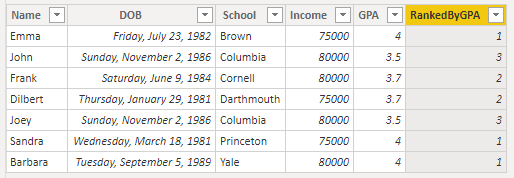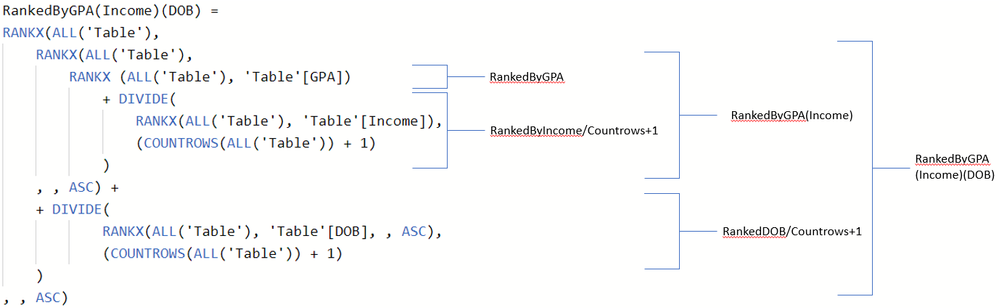cancel
Showing results for
Did you mean:## Breaking Ties in Rankings with RANKX Using Multiple Columns

There are situations in which you want to use a ranking of rows based on one column, but that column has non-unique values. Sometimes, there are other columns you want to use if two rows have the same value in the primary ranking column.  In this blog, I want to explain step by step how ranking based on multiple columns can help you solve this problem in DAX. The pattern used is something I picked up in this community, the focus in this blog is on the inner workings of that pattern.

I will use the following dataset in this post:

 Name School DOB Income GPA Emma Brown 7/23/1982 75,000 4 John Columbia 11/2/1986 80,000 3.5 Frank Cornell 6/9/1984 80,000 3.7 Dilbert Dartmouth 1/29/1981 75,000 3.7 Joey Columbia 11/2/1986 80,000 3.5 Sandra Princeton 3/18/1981 75,000 4 Barbara Yale 9/5/1989 80,000 4

Recently, I ran into the issue where I needed the show the top 2 rows in a calculated table. This is easily done by TOPN(<n>, <table>, <expression>), but what if I do this based on the dataset we have? Using N=2 on the GPA column, POWER BI returns 3 rows instead of 2:Standard ranking behavior and limits

If we want to rank the people in the dataset based on GPA, we can use a calculated column like this:

``RankedByGPA = RANKX('Table', 'Table'[GPA], , ,Dense) ``

The dataset will have an added column with the following values:Now, the current RankedByGPA column doesn’t really help us in our TOPN issue. If we would’ve used this column instead of the GPA column directly in the TOPN statement, we would have had the same result because there are 3 rows with the rank 1. So, breaking ties to the rescue!

Breaking ties with one additional column

In the case described above, we want to break ties in the RankedByGPA column. We are going to use the Income column for this, as a secondary ranking column. The DAX used for this is this:

``````RankedByGPA(Income) =
RANKX(ALL('Table'),
RANKX (ALL('Table'), 'Table'[GPA])
+ DIVIDE(
RANKX(ALL('Table'), 'Table'[Income]),
(COUNTROWS(ALL('Table')) + 1)
)
, , ASC)``````

So, let’s walk through this a bit, as it has some very interesting parts. First off, we see three (!) RANKX statements, which seems one too many. The reason lies in what is happening in the background. We start off by the outermost RANKX, which will use the full Table to evaluate. The expression it will use to rank the current row consists out of two parts which are summed (note the + before DIVIDE()):

``````1. RANKX (ALL('Table'), 'Table'[GPA])
2. DIVIDE(RANKX(ALL('Table'), 'Table'[Income]), (COUNTROWS(ALL('Table')) + 1)``````

Remember that this is a calculated column and is thus evaluated in a row context. To simplify, let’s look at the first row (Emma) of the dataset and walk through the evaluation. The first bit is ranking the current row based on the GPA column. In case of Emma, this will return 1, as we have seen in the previous examples. The second part ranks the current row based on Income and divides that by the count of rows + 1. In the case of Emma, the ranking of here income is evaluated to 2 as she is part of the group that makes 75,000 compared to the group that makes 80,000. This rank is then divided by the count of rows + 1 (which is 7 + 1). The returned value of the second bit is therefore 2 / 8 = 0.25

Now, remember that this 0.25 is added to the original ranking based on the GPA column, which was 1. The end value for the rows of Emma that is used to rank her against the other rows is thus 1.25. Let’s look at a different row and walkthrough the evaluation as well. In this case, let’s look at the last row, Barbara. She will be ranked based on the same sum of bits as Emma. Barbara has a GPA of 4 as well and will be ranked 1 in that regard. Her income however is ranked 1 (80,000) and that divided by the count of rows plus 1 equals (1 / (7 + 1) 😃 0.125. Barbara will be ranked based on a value of 1.125.

To see what values are used for the outer rank in the RankedByGPA(Income), I created columns of the in between steps in the following screenshots.Column RankedByGPA(Income) is based on the value of column OuterRankValued

As you can see, we have successfully broken some ties but there are still ties in there that we want to resolve as well. For example, our TOPN() calculated table from before would still return 3 rows if we wanted the top 2 rows based on RankedByGPA(Income). This is because there are two rows with a ranking of 2 and both will be returned aside from the row with ranking of 1.

Breaking ties on multiple columns

The next step is to alter our ranking in such a way that it will first look at GPA, and if these are the same then look at Income, and if these are also the same then look at Date of Birth (where older people are ranked higher than younger people). If you look at the above table, you might already notice that this will still return the exact same rank for John and Joey because these two happen to have the exact same values in these three columns.

To understand the solution, we are going to apply, you first need to understand why we used COUNTROWS(<table>) + 1 in the previous part. The sum of two rankings (which is what is basically happening) may never be bigger than the first ranking of the next item. Take Frank, for example. He has a rank of 2 based on GPA. The factor that we are going to add to this ranking must be smaller than 1 because otherwise the sum (= the final value that is ranked) would be larger than 3. Now, look at John who has a ranking of 3 in GPA. If he had no ties at all, his sum (=final value that is ranked) would be exactly 3. In that case, John will be ranked higher than Frank, although, based on GPA, Frank should always be ranked higher than John. Therefore, we divide the secondary ranking by a number that is the maximum possible ranking number (=the count of rows) plus 1. This will ensure the second bit will always be a value between 0 and 1.

That logic is important, as we are going to implement it now for not just a secondary column but for a tertiary column as well. Basically, we are taking RankedByGPA(Income) and put that into a new outer RANKX together with this:

``+ DIVIDE(RANKX(ALL('Table'), 'Table'[DOB]), (COUNTROWS(ALL('Table')) + 1)``

The total DAX looks like this;

``````RankedByGPA(Income)(DOB) =
RANKX(ALL('Table'),
RANKX(ALL('Table'),
RANKX (ALL('Table'), 'Table'[GPA])
+ DIVIDE(
RANKX(ALL('Table'), 'Table'[Income]),
(COUNTROWS(ALL('Table')) + 1)
)
, , ASC) +
+ DIVIDE(
RANKX(ALL('Table'), 'Table'[DOB], , ASC),
(COUNTROWS(ALL('Table')) + 1)
)
, , ASC)``````

I created an in-between column as well to explain the steps:RankedByGPA(Income)(DOB) is based on the sum of columns "RankedByGPA(Income)" and "RankedByDOB / Countrows +1"Mapping of the DAX to the different sub-columns

The fun part of RANKX is that it can be used on string values as well. Note that RANKX uses a descending order as default (rank 1 = the highest number). In our example dataset, we still have one tie on the rows of John and Joey. The only difference between these rows is their name, and we want to split these out as well. We wrap the entire DAX statement of RankedByGPA(Income)(DOB) in a new outer RANKX statement as we did with DOB:

``````RankedByGPA(Income)(DOB)(Name) =
RANKX(ALL('Table'),
RANKX(ALL('Table'),
RANKX(ALL('Table'),
RANKX (ALL('Table'), 'Table'[GPA])
+ DIVIDE(
RANKX(ALL('Table'), 'Table'[Income]),
(COUNTROWS(ALL('Table')) + 1)
)
, , ASC) +
+ DIVIDE(
RANKX(ALL('Table'), 'Table'[DOB], , ASC),
(COUNTROWS(ALL('Table')) + 1)
)
, , ASC) +
DIVIDE(
RANKX(ALL('Table'), 'Table'[Name], , ASC),
(COUNTROWS(ALL('Table')) + 1)
)
, , ASC)``````

Adding the in between column as well returns the following dataset:Well, and there you have it, we have ranked a dataset based on multiple columns without ANY ties. If you have more questions, please let me know!